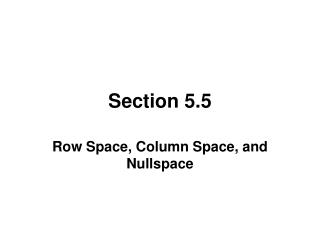DownloadDownload PresentationSection 5.5

# Section 5.5

Télécharger la présentation## Section 5.5

- - - - - - - - - - - - - - - - - - - - - - - - - - - E N D - - - - - - - - - - - - - - - - - - - - - - - - - - -
##### Presentation Transcript

1. Section 5.5 Row Space, Column Space, and Nullspace

2. ROW VECTORS For an m×n matrix the vectors in Rn formed from the rows of A are called the row vectors of A.

3. COLUMN VECTORS For the m×n matrix on the previous slide, the vectors in Rm formed from the columns of A are called the column vectors of A.

4. ROW SPACE, COLUMN SPACE, NULLSPACE If A is an m×n matrix, then the subspace of Rn spanned by the row vectors of A is called the row space of A, and the subspace of Rm spanned by the column vectors is called the column space of A. The solution space of the homogeneous system of equations Ax = 0, which is a subspace of Rn, is called the nullspace of A.

5. TWO QUESTIONS • What relationships exist between the solutions of a linear system Ax= b and the row space, column space, and nullspace of the matrix A? • What relationships exist among the row space, column space, and nullspace of a a matrix?

6. THEOREM Theorem 5.5.1: A system of linear equations Ax = b is consistent if and only if b is in the column space of A.

7. THEOREM Theorem 5.5.2: If x0 denotes any single solution of a consistent linear system Ax = b, and if v1, v2, ... , vk form a basis for the nullspace of A, then every solution of Ax = b can be expressed in the form x = x0 + c1v1 + c2v2 + ... + ckvk and, conversely, for all choices of scalars c1, c2, .. . , ck, the vector x in this formula is a solution of Ax = b.

8. PARTICULAR AND GENERAL SOLUTIONS • The vector x0 is called a particular solution of Ax = b. • The expression x0 + c1v1 + c2v2 + ... + ckvk is called the general solution of Ax = b. • The expression c1v1 + c2v2 + ... + ckvk is called the general solution of Ax = 0.

9. THEOREMS ON ROW SPACE AND NULLSPACE • Theorem 5.5.3: Elementary row operations do not change the nullspace of a matrix. • Theorem 5.5.4: Elementary row operations do not change the row space of a matrix.

10. A THEOREM ON ROW EQUIVALENT MATRICES Theorem 5.5.5: If A and B are row equivalent matrices, then: (a) A given set of column vectors of A is linearly independent if and only if the corresponding column vectors in B are linearly independent. (b) A given set of column vectors of A forms a basis for the column space of A if and only if the corresponding column vectors of B form a basis for the column space of B.

11. A ROW SPACE AND COLUMN SPACE THEOREM Theorem 5.5.6: If a matrix R is in row-echelon form, then the row vectors with the leading 1’s (the nonzero row vectors) form a basis for the row space of R, and the column vectors with the leading 1’s of the row vectors form a basis for the column space of R.